# On the generating graph of direct powers of a simple group

## Abstract

Let S be a nonabelian finite simple group and let n be an integer such that the direct product S n is 2-generated. Let Γ(S n) be the generating graph of S n and let Γ n (S) be the graph obtained from Γ(S n) by removing all isolated vertices. A recent result of Crestani and Lucchini states that Γ n (S) is connected, and in this note we investigate its diameter. A deep theorem of Breuer, Guralnick and Kantor implies that diam(Γ 1(S))=2, and we define Δ(S) to be the maximal n such that diam(Γ n (S))=2. We prove that Δ(S)≥2 for all S, which is best possible since Δ(A 5)=2, and we show that Δ(S) tends to infinity as |S| tends to infinity. Explicit upper and lower bounds are established for direct powers of alternating groups.

## Introduction

Let G be a finite group that can be generated by two elements and let Γ(G) be the generating graph of G; the vertices are the nontrivial elements of G, and two vertices are joined by an edge if and only if they generate G. This fascinating graph encodes many familiar generating properties. For example, G is said to be $$\frac {3}{2}$$-generated if every nontrivial element of G belongs to a generating pair; this is equivalent to the non-existence of isolated vertices in Γ(G). More generally, G has spread at least k if for any k nontrivial elements x 1,…,x k G, there exists yG such that G=〈x i ,y〉 for all i (this notion was introduced by Brenner and Wiegold  in the 1970s). Visibly, G has spread at least 2 if and only if Γ(G) is connected with diameter 2. Moreover, the graph-theoretic viewpoint suggests many new and natural questions. For instance, one can investigate the connectedness of Γ(G) (and subsequently its diameter), its (co-)clique and chromatic numbers, the existence of a Hamiltonian cycle in Γ(G), and so on.

Let S be a nonabelian finite simple group. It is well known that S can be generated by two elements, and there is a vast literature in this area. Indeed, many stronger results have been established in recent years. For example, a theorem of Guralnick and Kantor  states that S is $$\frac{3}{2}$$-generated (confirming a conjecture of Steinberg ), and a more recent result of the same authors (with Breuer) reveals that S has spread at least 2 (see ). In particular, it follows that the generating graph Γ(S) has diameter 2. The clique number ω(S) of Γ(S) (that is, the size of the largest complete subgraph) has also been investigated by several authors. In , Liebeck and Shalev prove that there is an absolute constant c>0 such that ω(S)≥cm(S) for any S, where m(S) is the minimal index of a proper subgroup of S. In , Blackburn shows that if n is a sufficiently large even integer which is indivisible by 4 then ω(A n )=2n−2 (and he also proves that this coincides with the chromatic number of Γ(A n )); see [7, 23] for related results. Another recent result reveals that Γ(S) contains a Hamiltonian cycle if |S| is sufficiently large (see ).

Let S n denote the direct product of n copies of S, and let δ(S) be the largest positive integer n such that S n is 2-generated. A formula of Philip Hall  states that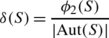where ϕ 2(S) denotes the number of ordered pairs (x,y) such that S=〈x,y〉. In particular, δ(S)=ℙ(S)|S|/|Out(S)| where ℙ(S) is the probability that two randomly chosen elements generate S. For example, δ(A 5)=19. By a striking theorem of Liebeck and Shalev  (see also [13, 19]), ℙ(S) tends to 1 as |S| tends to infinity, whence δ(S) also tends to infinity.

Let nδ(S) be a positive integer and consider the generating graph Γ(S n). If n≥2 then this graph contains isolated vertices, so following  we define Γ n (S) to be the graph obtained from Γ(S n) by removing all the isolated vertices. By [11, Theorem 1.1], Γ n (S) is connected, so it is natural to consider its diameter diam(Γ n (S)). Clearly, if n<δ(S) then diam(Γ n (S))≤diam(Γ n+1(S)), and [11, Theorem 1.2] states that diam(Γ n (S))≤4n−2. In addition, we note that there are examples where the diameter of Γ δ(S)(S) can be arbitrarily large. Indeed, [11, Theorem 1.3] states that if S=SL2(2p), where p is a prime, then diam(Γ δ(S)(S))≥2p−2−1 if p is sufficiently large.

We define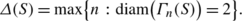(1)

Note that diam(Γ 1(S))=diam(Γ(S))=2 by the aforementioned theorem of Breuer, Guralnick and Kantor [5, Theorem 1.1], so Δ(S)≥1. Our main result is the following:

### Theorem 1

Let S be a nonabelian finite simple group. Then diam(Γ 2(S))=2, so Δ(S)≥2. Moreover, Δ(S) tends to infinity as |S| tends to infinity.

In Proposition 3.8, we show that Δ(A 5)=2, so the lower bound Δ(S)≥2 in Theorem 1 is best possible. The next theorem provides explicit bounds on Δ(S) when S is an alternating group.

### Theorem 2

Let S=A n be the alternating group of degree n≥5.

1. (i)

If n is odd then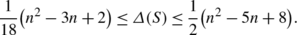2. (ii)

If n is even then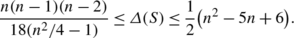### Remark 1

Note that the lower bound in part (ii) of Theorem 2 is linear in n. We refer the reader to Proposition 3.11 for a quadratic lower bound in the special case where n=2p with p an odd prime. It would be interesting to know whether or not a quadratic lower bound exists for all even n.

As one might expect, it appears to be much more difficult to obtain explicit bounds when S is a simple group of Lie type. However, the proof of Theorem 1 does provide the following lower bound on Δ(S). (Here r denotes the untwisted Lie rank of S, which is the rank of the ambient simple algebraic group.)

### Theorem 3

There exists an absolute constant c such that if S is a finite simple group of Lie type of rank r over $$\mathbb {F}_{q}$$, where q=p f with p a prime, then either S=Sp2r (2), or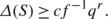### Remark 2

Let us make some remarks on the statement of Theorem 3:

1. (i)

The proof of Theorem 1 shows that we can take c=1/100 for the constant.

2. (ii)

The family of symplectic groups over the field of two elements is an anomaly. Indeed, it is well known that this family of groups has some unique generation properties. For example, this is the only infinite family of simple groups with exact spread two (the only other examples are A 5, A 6, and $$\varOmega _{8}^{+}(2)$$)—see [5, Corollary 1.3]. The proof of Proposition 5.5 shows that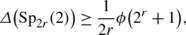where ϕ is the Euler totient function.

3. (iii)

It is difficult to determine the accuracy of the lower bound in Theorem 3, but it is clear that better bounds hold in certain cases. For example, if S=E 8(q), our proof yields Δ(S)≥cf −1 q 50 for some constant c (see Remark 5.3 for comments on the case where S is a classical group). Deriving good upper bounds on Δ(S) when S is a group of Lie type appears to be a difficult problem.

## Preliminaries

Let G be a finite group and let X be a subset of G. We say that X has the uniform spread two (UST) property if for any two nontrivial elements a,bG there exists an xX such that G=〈a,x〉=〈b,x〉. The main theorem of  states that if S is a nonabelian finite simple group then there is at least one conjugacy class in S with the UST property. The basic idea is to choose an element zS so that the set $$\mathcal{M}(z)$$ of maximal subgroups of S containing z is small and can be determined. As explained in [5, Sect. 2], the class C=z S has the UST property iffor all elements xS of prime order, where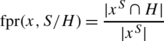(2)

denotes the fixed point ratio of x in its natural action on the set of right cosets S/H. In this way, upper bounds on fixed point ratios play an essential role in the proof of [5, Theorem 1.1].

For the remainder of this preliminary section, let S be a nonabelian finite simple group with automorphism group A=Aut(S). Define the integer Δ(S) as in (1).

### Proposition 2.1

Let C 1,…,C t be distinct A-classes in S with the UST property. Then Δ(S)≥t.

### Proof

This is entirely straightforward. Let x=(x 1,…,x t ) and y=(y 1,…,y t ) be distinct vertices in the graph Γ t (S). Then each x i ,y i is nontrivial, so there exists z i C i such that S=〈x i ,z i 〉=〈y i ,z i 〉. Set z=(z 1,…,z t )∈S t and consider L=〈x,z〉. By construction, π i (L)=S for all i, where π i denotes the ith projection map. Moreover, L is not contained in a diagonal subgroup of S t since z i and z j are not A-conjugate, for all ij. Therefore, L=S t. Similarly, 〈y,z〉=S t, and the result follows. □

Let X be a subset of S and let S # be the set of nontrivial elements in S. For a,bS # we defineand(3)

Then X has the UST property if and only if η(X)>0. By [5, Theorem 1.1], with the exception of a short list of known cases, there exists an S-class C=z S with η(C)≥1/3. Note that if C′=z A then C′ has the UST property and η(C′)=η(C).

The next result plays a key role in the proof of our main theorems.

### Proposition 2.2

Let $$C_{i} = z_{i}^{A}$$, 1≤it, be distinct A-classes in S with the UST property. For each i, set f i =η(C i ) and let k i be a positive integer such that(4)

where α(S)=max{|C A (x)| : xS #}. Then Δ(S)≥∑ i k i .

### Proof

We may assume t=1. Set C=C 1, z=z 1, f=f 1 and k=k 1. It suffices to prove the following claim:

Claim. If k satisfies (4) then any two vertices in Γ k (S) are connected to a vertex of the form (u 1,…,u k )∈Γ k (S), with u i C for all i.

We proceed by induction on k. Since C has the UST property and Γ 1(S)=Γ(S), the claim holds when k=1. Now assume k>1, and let (x 1,…,x k ) and (y 1,…,y k ) be vertices in Γ k (S). By induction, the vertices (x 1,…,x k−1) and (y 1,…,y k−1) in Γ k−1(S) are connected to a vertex (u 1,…,u k−1), where u i C for all i. In particular, the following conditions hold:

1. (i)

S=〈x i ,u i 〉=〈y i ,u i 〉 for all 1≤ik−1;

2. (ii)

For all distinct i,j∈{1,…,k−1}, the pairs (x i ,u i ) and (x j ,u j ) in S 2 are not A-conjugate, and nor are the pairs (y i ,u i ) and (y j ,u j ).

By definition, there are at least f|C| elements uC such that S=〈x k ,u〉=〈y k ,u〉. Fix i<k and suppose (x i ,u i ) is A-conjugate to (x k ,u) for some uC. Then $$x_{k}=x_{i}^{a}$$ for some aA and we deduce that there are precisely |C A (x i )| elements uC with this property. Similarly, if y i and y k are A-conjugate then there are exactly |C A (y i )| elements uC such that (y i ,u i ) is A-conjugate to (y k ,u). It follows that there are at least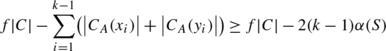choices for uC such that (u 1,…,u k−1,u) is connected to (x 1,…,x k ) and (y 1,…,y k ). The result now follows since our choice of k ensures that f|C|−2(k−1)α(S)>0. □

### Computational methods

Let S be a nonabelian finite simple group. In some specific cases (see Proposition 2.3, for example), we can use Magma  to find S-classes with the UST property. Here we briefly outline our methodology.

First, some notation. Let z be a nontrivial element of S and set C=z S. Our aim is to determine whether or not C has the UST property. As above, let $$\mathcal{M}(z)$$ denote the set of maximal subgroups of S containing z. For $$H \in\mathcal{M}(z)$$ and xS let fpr(x,S/H) be the fixed point ratio of x (see (2)), and let ℙ(x,z) be the probability that x and a randomly chosen element of C do not generate S. Note that(5)

We start with the standard permutation representation of S used by Magma (so for example, if S=PSL n (q) then this representation has degree (q n−1)/(q−1)). Using the commands MaximalSubgroups and ConjugacyClasses, we can determine the maximal subgroups of S which contain a conjugate of z; if H is such a subgroup then we can compute the fixed point ratio fpr(z,S/H)=|z SH|/|z S|, and we note that z belongs to exactly |S:H|⋅fpr(z,S/H) distinct S-conjugates of H. In this way, we can determine the subgroups in $$\mathcal{M}(z)$$, and we can subsequently compute σ(x,z) for any xS.

Now, if x 1,x 2S and σ(x i ,z)<1/2 for i=1,2 then (5) implies that ℙ(x i ,z)<1/2, so there is some element zC such that S=〈x 1,z〉=〈x 2,z〉. As previously remarked, if σ(x,z)<1/2 for all nontrivial xS (equivalently, for all xS of prime order), then C has the UST property and we are done. So let us assume that there are some nontrivial S-classes $$C_{i} = x_{i}^{S}$$, 1≤ik, with σ(x i ,z)≥1/2. For each x i we compute ℙ(x i ,z) as follows (see Breuer’s notes [4, p. 14]). First, we construct a set of (C S (z),C S (x i ))-double coset representatives. If rS is a representative then 〈x i ,z srt〉=〈x i ,z rt for all sC S (z), tC S (x i ), so we only need to test (non)generation for representatives. More precisely, if r 1,…,r are the representatives with $$S \neq \langle x_{i}, z^{r_{j}}\rangle$$ then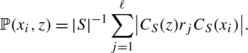Subsequently, we define m=max{ℙ(x i ,z) : 1≤ik}.

As before, if m<1/2 then C has the UST property, so let us assume m≥1/2. Note that ℙ(x,z)≤m for all xS #. Of course, if m=1 then there exists xS # such that S≠〈x,z〉 for all zC, so in this situation C does not have the UST property. Suppose m<1 and let y 1,y 2S be nontrivial elements such that σ(y 1,z)<1−m. Then ℙ(y 1,z)<1−m and ℙ(y 2,z)≤m, so there exists an element zC such that S=〈y i ,z〉 for i=1,2. Therefore, it remains to consider the conjugacy classes $$C_{i}=x_{i}^{S}$$, 1≤iv, such that σ(x i ,z)≥1−m. Fix i,j such that 1≤ijv, and let {y 1,…,y t } be a set of representatives of the C S (x i )-orbits on C j . For each y s we have to decide if there exists an element gS such that S=〈x i ,z g〉=〈y s ,z g〉. In practice, the existence of g can usually be established by testing a few randomly chosen elements, but exhaustive searches are required to prove non-existence. If we can always find such elements gS, for all possible i,j, then C=z S has the UST property.

By implementing the above procedure in Magma, we obtain the following result (note that the final statement follows immediately from Proposition 2.1).

### Proposition 2.3

Let S be one of the following simple groups: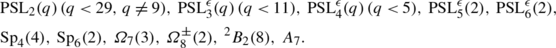Then there are at least two distinct A-classes in S with the UST property. In particular, in each case Δ(S)≥2.

### Remark 2.4

Let S be one of the groups in the statement of Proposition 2.3. In Table 1, we present an explicit list of S-classes with the UST property (we adopt the Atlas  labelling of classes). In all but three cases, the given list is complete. In the third column, we implicitly exclude the trivial class, and all classes of involutions. Note that there is a unique class in PSL2(9)≅A 6 with the UST property.

## Alternating groups

Let S=A n be the alternating group of degree n≥5. Recall that we define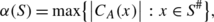where A is the automorphism group of S. The next lemma is easily checked (in general, |C A (x)| is maximal when x is a 3-cycle).

### Lemma 3.1

We haveFirst, we handle the alternating groups of small degree.

### Proposition 3.2

If n∈{5,6,7,8} then diam(Γ 2(A n ))=2.

### Proof

Set S=A n and A=Aut(S). If n=7 or 8 then Proposition 2.3 applies (note that A 8≅PSL4(2)), so let us assume n=5 or 6. In both cases, there is a unique A-class of elements in S with the UST property (comprising the elements of order 5 and 4, respectively). Set G=S×S and let VG be the set of vertices in Γ 2(S). First, assume n=5. Using Magma , it is easy to check that |V|=592 and V is the union of 16 G-classes, with representatives {x 1,…,x 16}. By a random search, for each x i and vV we can find an element wV such that G=〈x i ,w〉=〈v,w〉, hence diam(Γ 2(S))=2. The same method applies when n=6 (here |V|=3592 and V comprises 36 distinct G-classes). □

### Proposition 3.3

If n≥9 is odd then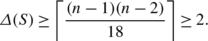### Proof

Let zS be an n-cycle and set C=z A. By [5, Proposition 6.7], C has the UST property with corresponding constant η(C)≥1/3 (see (3)). Now |C|=(n−1)! and α(S)=3(n−3)! (see Lemma 3.1), so the result follows from Proposition 2.2 (setting t=1, C 1=C and f 1=1/3). □

### Proposition 3.4

Suppose n≥10 is even and set ϵ=(2,n/2−1). Then### Proof

Let zS be an element with exactly two cycles, of lengths n/2±ϵ, where ϵ=(2,n/2−1). By [5, Proposition 6.3], C=z A has the UST property and η(C)≥1/3. Now |C|=n!/(n 2/4−ϵ 2), α(S)=3(n−3)! and once again the result follows from Proposition 2.2. □

### Corollary 3.5

The conclusion to Theorem 1 holds if S is an alternating group.

### Remark 3.6

The lower bounds obtained in Propositions 3.3 and 3.4 are respectively quadratic and linear in n. It is natural to ask whether or not a better lower bound can be obtained via Proposition 2.1. Let zS be an element such that C=z A has the UST property. Clearly, if z has four or more cycles then 〈z,(1,2,3)〉 is intransitive, so z has at most three cycles. In particular, if n is odd then z is either an n-cycle (which is the class used in the proof of Proposition 3.3), or z has exactly three cycles, so there are fewer than n 2 possibilities for C. This shows that by simply counting classes we cannot do better than a quadratic function in n. Similarly, if n is even then z has exactly two cycles, and so in this situation we cannot improve on a linear bound in n.

### Remark 3.7

It would be interesting to determine all the A-classes in S with the UST property, but this appears to be a difficult problem. Now, if n≥15 is odd and zS has cycle-shape (n 1,n 2,n 3), with n i ≥3 for all i, and (n i ,n j )=1 for ij, then one can show that z A has the UST property. Indeed, in this situation the analysis is simplified by the fact that z belongs to exactly three maximal subgroups of S, each of which is intransitive (see the proof of [17, Proposition 7.1]), so S=〈x,z〉 if and only if 〈x,z〉 is transitive. Similarly, if n≥10 is even and zS has cycle-shape (n 1,n 2), where n 1 and n 2 are coprime, then z A has the UST property.

Next we show that the lower bound Δ(S)≥2 in Theorem 1 is best possible. We note that S=A 5 is the only finite simple group for which the exact value of Δ(S) is currently known.

### Proposition 3.8

We have diam(Γ 3(A 5))=3, so Δ(A 5)=2.

### Proof

Let S=A 5, A=S 5 and set x=(x 1,x 2,x 3)∈S 3 and y=(y 1,y 2,y 3)∈S 3, where x i =(1,2,3) and y i =(1,3)(4,5) for all i. It is easy to check that x and y are non-isolated vertices in Γ(S 3). There are exactly 16 elements sS such that S=〈x 1,s〉=〈y 1,s〉, each of which is a 5-cycle, namely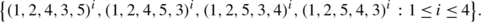It is straightforward to check that if z 1,z 2 and z 3 are distinct 5-cycles with this property then two of the z i are either C A (x 1)-conjugate or C A (y 1)-conjugate. We conclude that there is no zS 3 such that S 3=〈x,z〉=〈y,z〉, whence diam(Γ 3(S))≥3 and thus Δ(S)=2, as claimed.

To see that diam(Γ 3(S))=3, we have to work harder. Let z 1=(1,2,3,4,5), z 2=(1,2,3), and z 3=(1,2)(3,4). Set $$C_{i} = z_{i}^{A}$$ and note that S #=C 1C 2C 3. Let d i be the degree of z i in Γ(S) (that is, d i =|{xS : S=〈x,z i 〉}|) and set δ i =d i /|C A (z i )|. In addition, let α i (respectively, β i ,γ i ) be the number of elements z in C 1 (respectively, C 2, C 3) such that S=〈z,z i 〉. It is straightforward to check that these parameters take the following values:Note that (x 1,…,x n )∈Γ(S n) is non-isolated if and only if |{x r  : x r C i }|≤δ i for i=1,2,3. In particular, every vertex in (S #)3 is non-isolated. We also note the following:

(⋆):

Every pair of elements in C 1×C 2 generates S, while (u,v)∈C 1×C 1 is a pair of generators if and only if 〈u〉≠〈v〉.

Let x=(x 1,x 2,x 3) and y=(y 1,y 2,y 3) be any two vertices of Γ 3(S). To complete the proof of the proposition, it suffices to establish the following:

1. (i)

x is connected to two vertices u=(u 1,u 2,u 3) and $$v=(u_{1},u_{2},u_{3}')$$ in C 1×C 2×C 1 with $$\langle u_{3} \rangle \neq \langle u_{3}' \rangle$$;

2. (ii)

y is connected to a vertex in C 2×C 1×C 1;

3. (iii)

Every vertex in C 2×C 1×C 1 is connected to u or v.

First, consider (i). A vertex t=(t 1,t 2,t 3) in C 1×C 2×C 1 is connected to x if and only if S=〈x i ,t i 〉 for all i and (x 1,t 1) is not A-conjugate to (x 3,t 3) (since t is in C 1×C 2×C 1, (x 2,t 2) cannot be A-conjugate to (x 1,t 1) or (x 3,t 3)). As recorded in the above table, any nontrivial element in S generates with at least 16 elements in C 1 and with at least 6 elements in C 2. Hence, there are at least 16⋅6=96 choices for (t 1,t 2)∈C 1×C 2 such that S=〈x 1,t 1〉=〈x 2,t 2〉.

Let (u 1,u 2)∈C 1×C 2 be one of these choices and set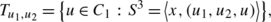so $$u \in T_{u_{1},u_{2}}$$ if and only if uC 1, S=〈x 3,u〉 and the pairs (x 1,u 1), (x 3,u) are not A-conjugate. If x 1 and x 3 are not A-conjugate then $$|T_{u_{1},u_{2}}| \geq16$$ (in this situation, $$u \in T_{u_{1},u_{2}}$$ if and only if uC 1 and S=〈x 3,u〉). On the other hand, if $$x_{3}=x_{1}^{\alpha}$$ for some αA then there are |C A (x 1)| choices for yC 1 such that (x 1,u 1) is A-conjugate to (x 3,y). Since x 3 generates with at least 16 elements in C 1, and |C A (x 1)|≤8, it follows that $$|T_{u_{1},u_{2}}|\geq8$$. Therefore, in every case we have $$|T_{u_{1},u_{2}}| \ge8$$, so we can find $$u_{3},u_{3}' \in T_{u_{1},u_{2}}$$ such that $$\langle u_{3}\rangle \neq \langle u_{3}'\rangle$$. This establishes (i), with u=(u 1,u 2,u 3) and $$v=(u_{1},u_{2},u_{3}')$$. By symmetry, (ii) also holds.

Finally, let us turn to (iii). Let t=(t 1,t 2,t 3) be a vertex in C 2×C 1×C 1. Then u is connected to t if and only if S=〈t i ,u i 〉 for all i, so (⋆) implies that u is connected to every vertex in the set {(t 1,t 2,t 3)∈C 2×C 1×C 1 : t 3∉〈u 3〉}. Similarly, v is connected to every vertex in the set $$\{ (t_{1},t_{2},t_{3}) \in C_{2} \times C_{1} \times C_{1} \,:\, t_{3} \notin \langle u_{3}' \rangle \}$$. Therefore, (iii) holds and the proof of the proposition is complete. □

We now consider upper bounds on Δ(A n ), with the aim of establishing Theorem 2.

### Proposition 3.9

Let S=A n with n≥6. Set ξ=6 if n is even, otherwise ξ=8. Then### Proof

Let s=(1,2,3)∈S and suppose σS is a permutation such that S=〈s,σ〉. Let fix(σ) be the set of fixed points of σ. Since 〈s,σ〉 is transitive, |fix(σ)|≤2 and σ has at most 3−|fix(σ)| cycles.

First, assume n is even. The following three cases arise:

1. (i)

If |fix(σ)|=0 then σ has exactly two cycles (since n is even).

2. (ii)

If |fix(σ)|=1 then σ is an (n−1)-cycle, and the fixed point is 1,2 or 3.

3. (iii)

If |fix(σ)|=2 then σ has to be an (n−2)-cycle, but there are no such permutations in S (since n is even).

Let k be the number of pairwise non-A-conjugate pairs (s,σ) such that S=〈s,σ〉 and |fix(σ)|=0. Then k=|F|/|C A (s)|, where F is the set of fixed-point-free permutations σS with S=〈s,σ〉. Note that FT, where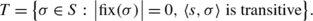As observed above, if 〈s,σ〉 is transitive and |fix(σ)|=0 then σ has exactly two cycles. Let 1<an/2 be an integer and let T a be the set of permutations σS of shape (a,na) such that 〈s,σ〉 is transitive. Note that $$T=\bigcup_{a=2}^{n/2}T_{a}$$. It is easy to check that if a<n/2 thenand similarly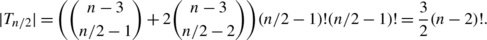We conclude thatIn particular, since |C A (s)|=3(n−3)!, it follows that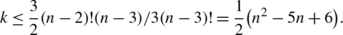Let x=(x 1,…,x k ,x k+1)∈Γ k+1(S) and y=(y 1,…,y k ,y k+1)∈Γ k+1(S), where x i =(1,2,3) and y i =(4,5,6) for all i. (Note that x (and also y) is a non-isolated vertex in Γ(S k+1); indeed, by definition of k there exists z=(z 1,…,z k+1)∈S k+1 with S k+1=〈x,z〉, where each z i with ik is a fixed-point-free permutation, and z k+1 has a single fixed point.) Since x i and y i have disjoint support, there is no element σS with fixed points such that S=〈x i ,σ〉=〈y i ,σ〉. In particular, if z=(z 1,…,z k+1)∈S k+1 and S k+1=〈x,z〉=〈y,z〉 then each z i must be fixed-point-free, but the definition of k implies that 〈x,z〉 is a proper subgroup of S k+1, a contradiction. We conclude thatA similar argument applies when n is odd. Here the following three cases arise:

1. (i)

If |fix(σ)|=0 then σ has at most three cycles, so either σ is an n-cycle, or σ has exactly three cycles.

2. (ii)

If |fix(σ)|=1 then σ has exactly two cycles, and the fixed point is 1,2 or 3.

3. (iii)

If |fix(σ)|=2 then σ is an (n−2)-cycle and fix(σ)={1,2},{1,3} or {2,3}.

Define k, F and T as before, and setNote that if n=3m and σ is a permutation with precisely three cycles of length m then 〈s,σ〉 is contained in a maximal imprimitive subgroup of S of type S 3S m .

In order to define certain subsets of P and T, setand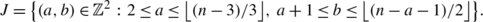For aI, let P a,a (respectively, T a,a ) be the set of permutations σS of shape (a,a,n−2a) such that 〈s,σ〉 is primitive (respectively, transitive). Similarly, for (a,b)∈J let P a,b (respectively, T a,b ) be the set of permutations σS of shape (a,b,nab) such that 〈s,σ〉 is primitive (respectively, transitive). Note thatIt is straightforward to check that |T 0,0|=(n−1)! andif aI is non-zero. Similarly, if (a,b)∈J thenand we calculate that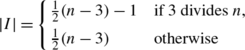andTherefore,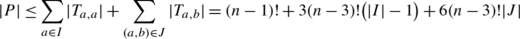and thussince |C A (s)|=3(n−3)!. We now complete the argument as in the n even case to getas required. □

By combining Propositions 3.3 and 3.9, we obtain the following quadratic bounds on Δ(S) when S is an alternating group of odd degree.

### Corollary 3.10

If n≥9 is odd thenThis completes the proof of Theorem 2. Note that when n is even, Proposition 3.4 provides a linear lower bound on Δ(A n ). As the next result demonstrates, a quadratic lower bound can be established in some special cases.

### Proposition 3.11

Suppose S=A n , where n=2p with p>3 a prime. Then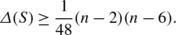### Proof

Let C h be the S n -class of permutations in S of shape (a,2pa), where a=2h+1 is odd and 1≤h≤(p−3)/2. Let σ 1,σ 2S be nontrivial permutations and setand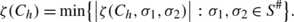Note that ζ(C h )=|C h |η(C h ) (see (3)). Since a is odd and ap−2, it follows that the integers a and 2pa are coprime, whence S=〈z,σ i 〉 if and only if 〈z,σ i 〉 is transitive. We claim that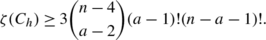(6)

Clearly, in order to establish this lower bound, we may assume that each σ i has prime order. Write σ 1=σ 1,1σ 1,r and σ 2=σ 2,1σ 2,s as disjoint cycles, where each σ i,j has prime length. There are two cases to consider.

First, suppose σ 1,i =σ 2,j for some i,j. In this case,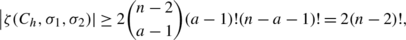and the desired bound follows.

Now assume σ 1,i σ 2,j for all i,j. Given πS n , let supp(π) denote the support of π. Then there exists {x 1,x 2}∈supp(σ 1,1) and {y 1,y 2}∈supp(σ 2,1) such that either {x 1,x 2}∩{y 1,y 2}=∅ or {x 1,x 2}∩{y 1,y 2}={x 1}={y 1}. In the former situation, we have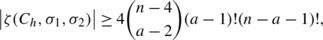while in the latter case we calculate thatThis establishes the lower bound in (6).

Now Lemma 3.1 states that α(S)=3(n−3)!, so Proposition 2.2 yieldsFinally, by using the lower bound on ζ(C h ) in (6), we calculate that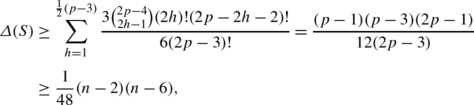as required. □

### Proposition 4.1

The conclusion to Theorem 1 holds if S is a sporadic group.

### Proof

Let S be a sporadic simple group and let zS be an element in the S-class recorded in the second column of [5, Table 7]. Then [5, Lemma 6.1] states that C=z A has the UST property with corresponding constant η(C)≥1/3 (see (3)) and the desired bound Δ(S)≥2 quickly follows from Proposition 2.2. (Note that α(S) can be easily calculated from the character table of S – see .) For example, if S=M11 and we take C=z S to be the class 11A then |C|=720, η(C)=1/3, α(S)=48 and we deduce that Δ(S)≥3. The remaining cases are entirely similar. □

## Classical groups

In this section, we establish Theorems 1 and 3 for classical groups.

### Proposition 5.1

Let S be a finite simple classical group over $$\mathbb {F}_{q}$$ with automorphism group A. Write q=p f where p is a prime. Then α(S)≤Λ, where Λ is given in Table  2.

### Proof

Let G=Inndiag(S) be the group of inner-diagonal automorphisms of S. Define d and Q as in Table 2. Let xS be an element of prime order and define the integer ν(x) as in [8, Definition 3.16] (so ν(x) is the codimension of the largest eigenspace of a lift $$\hat{x} \in\mathrm{GL}(V)$$ of x on $$V \otimes\bar{\mathbb {F}}_{q}$$, where V is the natural S-module). Note that C A (x)≤C A (x i) for all i≥1, so we only need to consider elements of prime order.

First, assume S=PSL n (q). If n=2 then it is easy to check that |C G (x)|≤d(q+1) and thus |C A (x)|≤df(q+1) since |A:G|=f. If n≥3 then [8, Corollary 3.38] implies that $$|x^{G}|>\frac{1}{2}q^{2n-2}$$, so $$|C_{G}(x)|<2q^{n^{2}-2n+1}$$ (since $$|G|<q^{n^{2}-1}$$), and we deduce that $$|C_{A}(x)|<4fq^{n^{2}-2n+1}$$ since |A:G|=2f. An entirely similar argument applies when S is a unitary group.

Next suppose S=PSp n (q)′. First, observe that if x is a transvection thenNow, if n=4 and q is even then it is easy to check that |C G (x)| is maximal when x is a transvection, so |C G (x)|≤q 4(q 2−1) and thus |C A (x)|≤fq 4(q 2−1) (note that a transvection is not centralized by a graph automorphism of S). On the other hand, if n=4 and q is odd then |C G (x)|≤2|Sp2(q)|2=2q 2(q 2−1)2, so |C A (x)|≤2fq 2(q 2−1)2. Now assume n≥6. If x is not a transvection then ν(x)≥2 and thus [8, Corollary 3.38] yields $$|x^{G}|>\frac{1}{4}Q^{-1}q^{2n-4}$$. We deduce that transvections have the largest centralizers, so |C G (x)|<q n(n−1)/2 and thus |C A (x)|<fq n(n−1)/2.

Finally, let us assume S is an orthogonal group. If n is even and (n,ϵ)≠(8,+) then we apply [8, Corollary 3.38] as before, noting that ν(x)≥2 since xS. Similarly, if (n,ϵ)=(8,+) then |x G| is minimal when x is a long root element, in which case |C G (x)|=q 12(q 2−1)3 and thus |C A (x)|<6fq 18 since |A:G|=6f. Finally, suppose n is odd. We claim that $$|C_{G}(x)|<2q^{n^{2}/2-3n/2+1}$$. If ν(x)≥2 then [8, Corollary 3.38] gives $$|x^{G}|>\frac{1}{4}Q^{-1}q^{2n-6}$$ and the claim follows. On the other hand, if ν(x)=1 then x is an involution and $$|C_{G}(x)| \le2|\mathrm{SO}_{n-1}^{-}(q)|<2q^{n^{2}/2-3n/2+1}$$. This justifies the claim, and we conclude that $$|C_{A}(x)|<4fq^{n^{2}/2-3n/2+1}$$. □

### Proposition 5.2

The conclusions to Theorems 1 and 3 hold when S=PSL n (q).

### Proof

First, assume n=2. If q<29 (and q≠9) then Proposition 2.3 applies, so we may assume q≥29 (note that PSL2(9)≅A 6, so Proposition 3.2 applies in this case). Then Proposition 5.1 states that α(S)≤df(q+1), and [5, Proposition 5.24] provides an A-class C with η(C)≥1/3 (see (3)) and |C|≥q(q−1). Therefore, by applying Proposition 2.2, we deduce that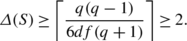Now consider the general case n≥3. Here $$\alpha (S)\le4fq^{n^{2}-2n+1}$$ and, by inspecting [5, Table 5 and Sect. 5.12], we see that there exists an A-class C of regular semisimple elements with η(C)≥1/3. Therefore, $$|C| > \frac{1}{2}q^{n(n-1)}$$, and thus Proposition 2.2 implies that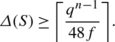This bound is sufficient unless n=3 (with q<11), n=4 (with q<5) or (n,q)=(5,2), (6,2). In each of these exceptional cases, Proposition 2.3 applies. □

### Remark 5.3

The lower bounds obtained in the proof of Proposition 5.2 are good enough to establish Theorems 1 and 3 when S=PSL n (q), but they can be improved. For example, suppose S=PSL n (q) with n≥12. Let zS be a regular semisimple element which lifts to an element $$\hat{z} \in\mathrm{SL}_{n}(q)$$ of order lcm(q e−1,q ne−1)/(q−1), where(Here $$\hat{z}$$ preserves a decomposition V=UW of the natural SL n (q)-module V, acting irreducibly on both U and W, with dimU=e. Also note that (e,ne)=1.) By [5, Proposition 5.23], the S-class C=z S has the UST property, with η(C)≥1/2. Indeed, $$\hat{z}$$ is contained in exactly two maximal subgroups of SL n (q); namely, the stabilizers of the subspaces U and W. Let N be the number of distinct A-classes in S containing regular semisimple elements of this form. By considering the possible eigenvalues of $$\hat {z}$$ (in $$\bar{\mathbb{F}}_{q}$$), it is easy to see that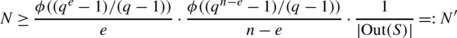where ϕ is the Euler totient function. Therefore, by arguing as in the proof of Proposition 5.2, using Proposition 2.2, we obtain a better lower bound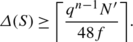By carefully inspecting [5, Sect. 5], similar lower bounds on the number of A-classes with the UST property can be derived for any classical group S. However, we do not have a good upper bound on Δ(S) when S is a group of Lie type, so the accuracy of the improved lower bounds is difficult to determine.

### Proposition 5.4

The conclusions to Theorems 1 and 3 hold when S=PSU n (q).

### Proof

If n=3 (and q<11), n=4 (and q<5) or (n,q)=(5,2),(6,2) then Proposition 2.3 applies, so let us assume otherwise. By inspecting [5, Propositions 5.21, 5.22], it follows that there exists an A-class C with η(C)≥1/3 and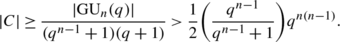As before, by applying Proposition 2.2 and the upper bound on α(S) recorded in Table 2, we deduce that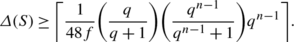This bound is sufficient unless (n,q)=(7,2). Here(see [5, Proposition 5.21]), and, by applying Proposition 2.2, we deduce that Δ(S)≥6. □

### Proposition 5.5

The conclusions to Theorems 1 and 3 hold when S=PSp n (q)′.

### Proof

First, assume n=4. If 2<q<5 then Proposition 2.3 applies (see Proposition 3.2 for the case q=2, since PSp4(2)′≅A 6). Suppose q≥5. By [5, Propositions 5.8, 5.12], there exists an A-class C with |C|≥q 4(q 2−1)2 and η(C)≥1/3. In the usual way, via Propositions 2.2 and 5.1, we deduce that Δ(S)≥⌈q 2/12f⌉ and the result follows.

Now assume n≥6. The case q=2 requires special attention; indeed, this is one of the exceptional cases recorded in [5, Theorem 1.1]. By [5, Proposition 5.8], if zS is an irreducible element of order 2n/2+1 then C=z S has the UST property. Now there are precisely ϕ(2n/2+1)/n distinct A-classes of such elements, so Proposition 2.1 implies that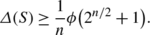It is easy to check that this bound implies that Δ(S)≥2 when 8≤n≤14. In general, if we write $$2^{n/2}+1=\prod_{i}p_{i}^{a_{i}}$$, where the p i are distinct primes, then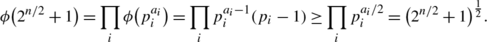The subsequent bound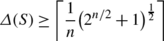is sufficient for all n≥16. The case (n,q)=(6,2) is covered by Proposition 2.3.

Finally, let us assume n≥6 and q≥3. By inspecting [5, Propositions 5.8, 5.10, 5.12], we see that there exists an A-class C with η(C)≥1/3 and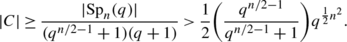The desired result now follows in the usual way via Proposition 2.2, using the upper bound on α(S) given in Table 2. □

### Proposition 5.6

The conclusions to Theorems 1 and 3 hold when $$S=\mathrm{P\varOmega }_{n}^{\epsilon }(q)$$.

### Proof

We may assume n≥7. First, suppose (n,ϵ)=(8,+). If q=2 then Proposition 2.3 applies. Now if q=3 then [5, Table 3] indicates that there is an A-class C=z A in S with the UST property, where |z|=20 and η(C)≥2(1−195/455)=67/455 (here z preserves an orthogonal decomposition 8+=4⊥4 of the natural S-module into nondegenerate 4-spaces of minus type). We calculate thatand thus Proposition 2.2 implies that Δ(S)≥34. Similarly, if q=4 then [5, Table 3] provides an A-class C=z A in S with |z|=65 (preserving a decomposition 8+=2⊥6), η(C)≥1/3 and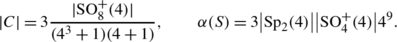Once again, the desired result follows by applying Proposition 2.2.

Next assume (n,ϵ)=(8,+) and q≥5. By [5, Lemma 5.15], there is an A-class C with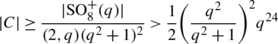and η(C)≥1/3. According to Proposition 5.1, we have α(S)≤6fq 18, so Proposition 2.2 implies that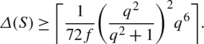It is easy to check that this bound is always sufficient.

For the remainder we may assume that (n,ϵ)≠(8,+). We first deal with the case where n is odd and q=3. Note that if n≡1(mod4) then S is one of the exceptional cases recorded in [5, Theorem 1.1]. In view of Proposition 2.3, we may assume that n≥9. By [5, Propositions 5.7, 5.19], there is an A-class C in S such thatand η(C)≥1/3. By combining this lower bound with the upper bound on α(S) given in Proposition 5.1, we quickly deduce that Proposition 2.2 yieldsand this bound is sufficient unless n=9. Here $$\alpha (S)=2|\mathrm{SO}_{8}^{+}(3)|$$ and there is an A-class C such that η(C)≥1/3 and |C|=|SO9(3)|/82 (see [5, Proposition 5.7]). In the usual way, via Proposition 2.2, we deduce that Δ(S)≥7.

The remaining cases are very similar. By inspecting [5, Sections 5.6, 5.8, 5.9], it follows that there is an A-class C=z A in S of regular semisimple elements such that η(C)≥1/3 and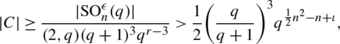where r denotes the rank of S, and ι=1/2 if n is odd, otherwise ι=0. The result now follows in the usual way, via Propositions 5.1 and 2.2. For example, if n is even then we deduce that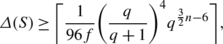and this bound is sufficient unless (n,q)=(8,2), which is one of the cases handled in Proposition 2.3. □

## Exceptional groups

Here we complete the proof of Theorems 1 and 3 by dealing with the exceptional groups of Lie type.

### Proposition 6.1

Let S be a finite simple exceptional group of Lie type over $$\mathbb {F}_{q}$$, where q=p f with p a prime. Then α(S)≤Λ, where Λ is given in Table  3.

### Proof

Detailed information on the conjugacy classes in S can be found in the literature, and the result follows by inspecting the relevant lists of conjugacy class sizes. For example, let S=E 8(q) and let xS be an element of prime order. The sizes of the unipotent classes in S are conveniently listed in [20, Table 22.2.1], and it is easy to see that |C S (x)|≤q 57|E 7(q)|, with equality if and only if x is a long root element. For semisimple x, the possibilities for |C S (x)| are listed in  and it is easy to check that |C S (x)|≤|E 7(q)||SL2(q)|. Since |A:S|=f (where A=Aut(S)) we conclude that α(S)≤fq 57|E 7(q)| as claimed. The other cases are very similar and we leave the reader to check the details. (See [15, 20] for the sizes of conjugacy classes in E 7(q) and $$E_{6}^{\epsilon }(q)$$; [24, 26] for F 4(q), [9, 14] for G 2(q),  for 2 F 4(q),  for 2 G 2(q),  for 2 B 2(q) and [12, 27] for 3 D 4(q).) □

### Proposition 6.2

The conclusion to Theorem 1 holds when S is a finite simple exceptional group of Lie type.

### Proof

By [5, Lemma 6.2], there exists an A-class C=z A of regular semisimple elements in S with η(C)≥1/3 (see [17, Propositions 6.1, 6.2]). The desired result now follows via Proposition 2.2, using the upper bound on α(S) in Proposition 6.1 and a suitable lower bound on |C|. For example, suppose S=G 2(q)′. If q=2 then S≅PSU3(3) and thus Proposition 5.4 applies. For q>2 we have $$|C|>\frac {1}{2}Q^{-2}q^{12}$$ (where Q=(q+1)/q as before) and α(S)<2fq 8, so Proposition 2.2 yields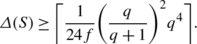It is easy to check that this bound gives the desired result. Similarly, if S=2 G 2(q)′ (so that q=32m+1 for some positive integer m) then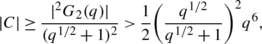and we deduce that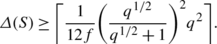This bound is sufficient if q>3, while Proposition 5.2 applies if q=3 (since S≅PSL2(8)). The remaining cases are entirely similar. □

This completes the proof of Theorems 1 and 3.

## References

1. Blackburn, S.R.: Sets of permutations that generate the symmetric group pairwise. J. Comb. Theory, Ser. A 113, 1572–1581 (2006)

2. Bosma, W., Cannon, J., Playoust, C.: The Magma algebra system I: the user language. J. Symb. Comput. 24, 235–265 (1997)

3. Brenner, J.L., Wiegold, J.: Two-generator groups I. Mich. Math. J. 22, 53–64 (1975)

4. Breuer, T.: GAP computations concerning the probabilistic generation of finite simple groups. Preprint arXiv:0710.3267

5. Breuer, T., Guralnick, R.M., Kantor, W.M.: Probabilistic generation of finite simple groups, II. J. Algebra 320, 443–494 (2008)

6. Breuer, T., Guralnick, R.M., Lucchini, A., Maróti, A., Nagy, G.P.: Hamiltonian cycles in the generating graph of finite groups. Bull. Lond. Math. Soc. 42, 621–633 (2010)

7. Britnell, J.R., Evseev, A., Guralnick, R.M., Holmes, P.E., Maróti, A.: Sets of element that pairwise generate a linear group. J. Comb. Theory, Ser. A 115, 442–465 (2008)

8. Burness, T.C.: Fixed point ratios in actions of finite classical groups, II. J. Algebra 309, 80–138 (2007)

9. Chang, B.: The conjugate classes of Chevalley groups of type (G 2). J. Algebra 9, 190–211 (1968)

10. Conway, J., Curtis, R., Norton, S., Parker, R., Wilson, R.: Atlas of Finite Groups. Oxford University Press, London (1985)

11. Crestani, E., Lucchini, A.: The non-isolated vertices in the generating graph of direct powers of simple groups. J. Alg. Combin. (2012). doi:10.1007/s10801-012-0365-1

12. Deriziotis, D.I., Michler, G.: Character table and blocks of the finite simple triality groups 3 D 4(q). Trans. Am. Math. Soc. 303, 39–70 (1987)

13. Dixon, J.D.: The probability of generating the symmetric group. Math. Z. 110, 199–205 (1969)

14. Enomoto, H.: The conjugacy classes of Chevalley groups of type (G 2) over finite fields of characteristic 2 or 3. J. Fac. Sci. Univ. Tokyo 16, 497–512 (1970)

15. Fleischmann, P., Janiszczak, I.: The semisimple conjugacy classes of finite groups of Lie type E 6 and E 7. Commun. Algebra 21, 93–161 (1993)

16. Fleischmann, P., Janiszczak, I.: The semisimple conjugacy classes and the generic class number of the finite simple groups of Lie type E 8. Commun. Algebra 22, 2221–2303 (1994)

17. Guralnick, R.M., Kantor, W.M.: Probabilistic generation of finite simple groups. J. Algebra 234, 743–792 (2000)

18. Hall, P.: The Eulerian functions of a group. Q. J. Math. 7, 134–151 (1936)

19. Kantor, W.M., Lubotzky, A.: The probability of generating a finite classical group. Geom. Dedic. 36, 67–87 (1990)

20. Liebeck, M.W., Seitz, G.M.: Unipotent and Nilpotent Classes in Simple Algebraic Groups and Lie Algebras. Monographs and Surveys Series, vol. 180. Am. Math. Soc., Providence (2012)

21. Liebeck, M.W., Shalev, A.: The probability of generating a finite simple group. Geom. Dedic. 56, 103–113 (1995)

22. Liebeck, M.W., Shalev, A.: Simple groups, probabilistic methods, and a conjecture of Kantor and Lubotzky. J. Algebra 184, 31–57 (1996)

23. Lucchini, A., Maróti, A.: On the clique number of the generating graph of a finite group. Proc. Am. Math. Soc. 137, 3207–3217 (2009)

24. Shinoda, K.: The conjugacy classes of Chevalley groups of type (F 4) over finite fields of characteristic 2. J. Fac. Sci. Univ. Tokyo 21, 133–159 (1974)

25. Shinoda, K.: The conjugacy classes of the finite Ree groups of type (F 4). J. Fac. Sci. Univ. Tokyo 22, 1–15 (1975)

26. Shoji, T.: The conjugacy classes of Chevalley groups of type (F 4) over finite fields of characteristic p≠2. J. Fac. Sci. Univ. Tokyo 21, 1–17 (1974)

27. Spaltenstein, N.: Caractères unipotents de $${}^{3}D_{4}(\mathbb {F}_{q})$$. Comment. Math. Helv. 57, 676–691 (1982)

28. Steinberg, R.: Generators for simple groups. Can. J. Math. 14, 277–283 (1962)

29. Suzuki, M.: On a class of doubly transitive groups. Ann. Math. 75, 105–145 (1962)

30. Ward, H.N.: On Ree’s series of simple groups. Trans. Am. Math. Soc. 121, 62–89 (1966)

## Acknowledgements

Burness is supported by EPSRC grant EP/I019545/1, and he thanks the Dipartimento di Matematica at the Università degli Studi di Padova for their generous hospitality. Crestani is supported by a grant from Fondazione Ing. Aldo Gini. Both authors thank Andrea Lucchini and Pablo Spiga for helpful comments.

## Author information

Authors

### Corresponding author

Correspondence to Timothy C. Burness.

## Rights and permissions

Reprints and Permissions

Burness, T.C., Crestani, E. On the generating graph of direct powers of a simple group. J Algebr Comb 38, 329–350 (2013). https://doi.org/10.1007/s10801-012-0405-x

• Accepted:

• Published:

• Issue Date:

• DOI: https://doi.org/10.1007/s10801-012-0405-x

### Keywords

• Finite simple groups
• Generating graph
• Diameter